Introduction to polymers

Start this free course now. Just create an account and sign in. Enrol and complete the course for a free statement of participation or digital badge if available.

Free course

2.6 Molecular mass distribution

In Figure 28 the peak (Mp) is at about 40 000. Number average (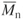) and weight average (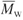) molecular masses fall below and above the peak value. Determined using high temperature GPC

Chain molecules give rise to types of structure which are not formed in small molecules, and they exhibit molecular mass variations which are critical not only for their properties but also for processing into shape. In general, commercial polymers do not consist of assemblies of chains of constant length but rather a distribution of lengths, so that statistical techniques of analysis are needed to characterize them. The spectrum of molecular masses for a typical HDPE is shown in Figure 28, with M varying from M=200 through a peak at about 40 000 to over a million. Such a distribution can be described by average molecular masses, of which the most important are the number-average molecular massthe weight-average molecular massand the z-average molecular masswhere N i and W i are the number and weight of chains of molecular mass M respectively. Since Wi = NiMi, Equation (5) can be rewritten asand Equation (6) becomesEach measure of molecular mass gives a different emphasis.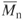emphasises the smaller molecules present whereas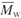and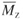emphasise the larger molecules. It is clear therefore that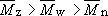as shown in Figure 28. A simple example suffices to demonstrate the point. An equimolecular (i.e. equimolar) mixture of samples of molecular mass 50 000 and 100 000 will have the following average values: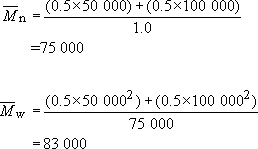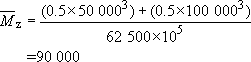The breadth of the distribution is usually defined by the dispersion, which is the simple ratio of weight- to number-average molecular masses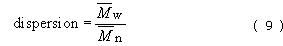which is unity for a monodisperse polymer but is usually greater than unity. High density polyethylenes have values from 6 to 12 while LDPE is usually much broader in distribution (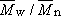up to ca. 30). New metallocene polyolefins, however, have low dispersions of about 2.5. In polymers like nylon it is also much sharper, with values in the region of 2.0.

The molecular mass distribution (MMD) is important for many physical properties of the polymer and is best expressed by a complete curve as shown in Figure 28, obtained directly by a method known as gel permeation chromatography (GPC) (Box 5).

Single-point averages can be obtained by other means such as osmometry (Mn), light scattering (Mw) or ultracentrifugation (Mz). Dilute solution viscometry gives a direct measure of the viscosity-average molecular mass Mv, which usually lies between Mn and Mw. It is defined by the equationwhere a is a constant in the equation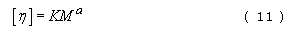[η] is the intrinsic viscosity of a polymer in solution and K is another constant characteristic of the solution. K usually lies between 0.5 × 10−4 and 5 × 10−4, while a typically lies in the range 0.6–0.8.

Self assessment question 4

To improve processing properties, an equal weight of low molecular mass nylon 6 (degree of polymerization n = 100) is blended with a moulding grade of nylon 6 (n = 500). Assuming both materials are monodisperse, what will be

1. the weight-average molecular mass,

2. the number-average molecular mass,

3. the dispersion of the blend?

From Table 3, the molecular masses of the additive (1) and the base (2) can be calculated knowing n, the degree of polymerization: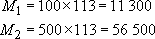(a)Now from Equation (5),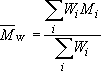so for equal weights of each component.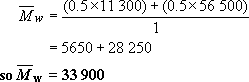(b)Now Ni = Wi/Mi so that the number of moles of each component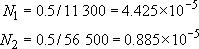So from Equation (4),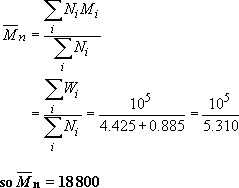(c) Hence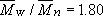Box 5 Gel permeation chromatography (GPC)

GPC is a technique for determining the molecular mass distribution of a polymer by analysis of a solution of the polymer concerned. It is normally a rapid and accurate way of determining the MMD compared with older methods such as osmometry or light scattering. Moreover, it gives the whole distribution in one pass, while the other methods give only single point averages. Light scattering, for example, only yields the weight-average molecular mass. When the complete MMD is available from GPC, any average molecular mass can be computed simply and easily.

But since a solution is the basis for analysis using GPC, the technique is limited to thermoplastic, uncrosslinked polymers. Polymers vary widely in their ability to form solutions. Some, such as polystyrene and polycarbonate are soluble in a wide selection of organic media. Tetrahydrofuran or THF is a useful solvent for their GPC analysis. Many other plastics materials, however, are quite insoluble in the range of solvents commonly available. They include the polyolefins, PE and PP, as well as PET and other engineering polymers with high TgS. GPC in a high boiling point solvent such as decalin (Tb = 169 °C) is often used for such intractable materials.

So what is the basis of GPC? The method involves passing the polymer solution down a column containing gel spheres into which the smallest chains will diffuse most easily, the largest with extreme difficulty. The latter are thus the first to be passed out at the bottom of the column, at low elution counts, while the smallest chains diffuse out last of all. The polymer concentration is measured in the eluate, so giving an estimate of the MMD. The method demands calibration with standard samples, usually monodisperse polystyrene of various molecular masses (Figure 29).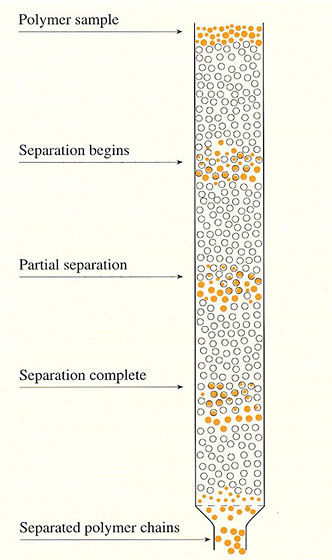Figure 29

When is GPC used? Basically, whenever a user wants to check the MMD of a polymer specimen against, say, a virgin or unused sample. The user may be interested in checking whether the correct grade of polymer has been used, for example, in a plastic moulding, or alternatively, the effects of chain degradation.